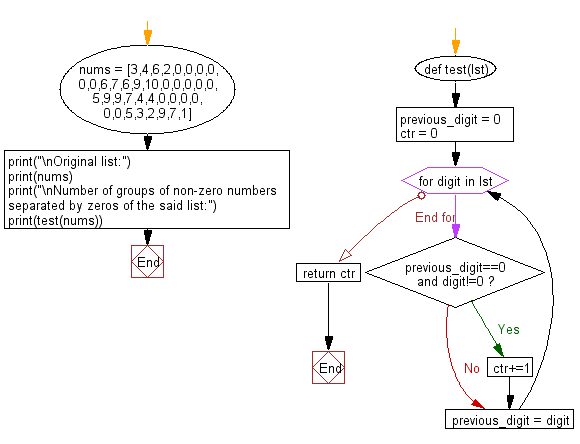﻿ Python: Count the number of groups of non-zero numbers separated by zeros of a given list of numbers - w3resource# Python: Count the number of groups of non-zero numbers separated by zeros of a given list of numbers

## Python List: Exercise - 209 with Solution

Write a Python program to count the number of groups of non-zero numbers separated by zeros of a given list of numbers.

Sample Solution:

Python Code:

``````def test(lst):
previous_digit = 0
ctr = 0
for digit in lst:
if previous_digit==0 and digit!=0:
ctr+=1
previous_digit = digit
return ctr

nums = [3,4,6,2,0,0,0,0,0,0,6,7,6,9,10,0,0,0,0,0,5,9,9,7,4,4,0,0,0,0,0,0,5,3,2,9,7,1]

print("\nOriginal list:")
print(nums)
print("\nNumber of groups of non-zero numbers separated by zeros of the said list:")
print(test(nums))
```
```

Sample Output:

```Original list:
[3, 4, 6, 2, 0, 0, 0, 0, 0, 0, 6, 7, 6, 9, 10, 0, 0, 0, 0, 0, 5, 9, 9, 7, 4, 4, 0, 0, 0, 0, 0, 0, 5, 3, 2, 9, 7, 1]

Number of groups of non-zero numbers separated by zeros of the said list:
4
```

Flowchart:## Visualize Python code execution:

The following tool visualize what the computer is doing step-by-step as it executes the said program:

Python Code Editor:

Have another way to solve this solution? Contribute your code (and comments) through Disqus.

What is the difficulty level of this exercise?

Test your Python skills with w3resource's quiz

﻿

## Python: Tips of the Day

```print(2_000_000)
```2000000